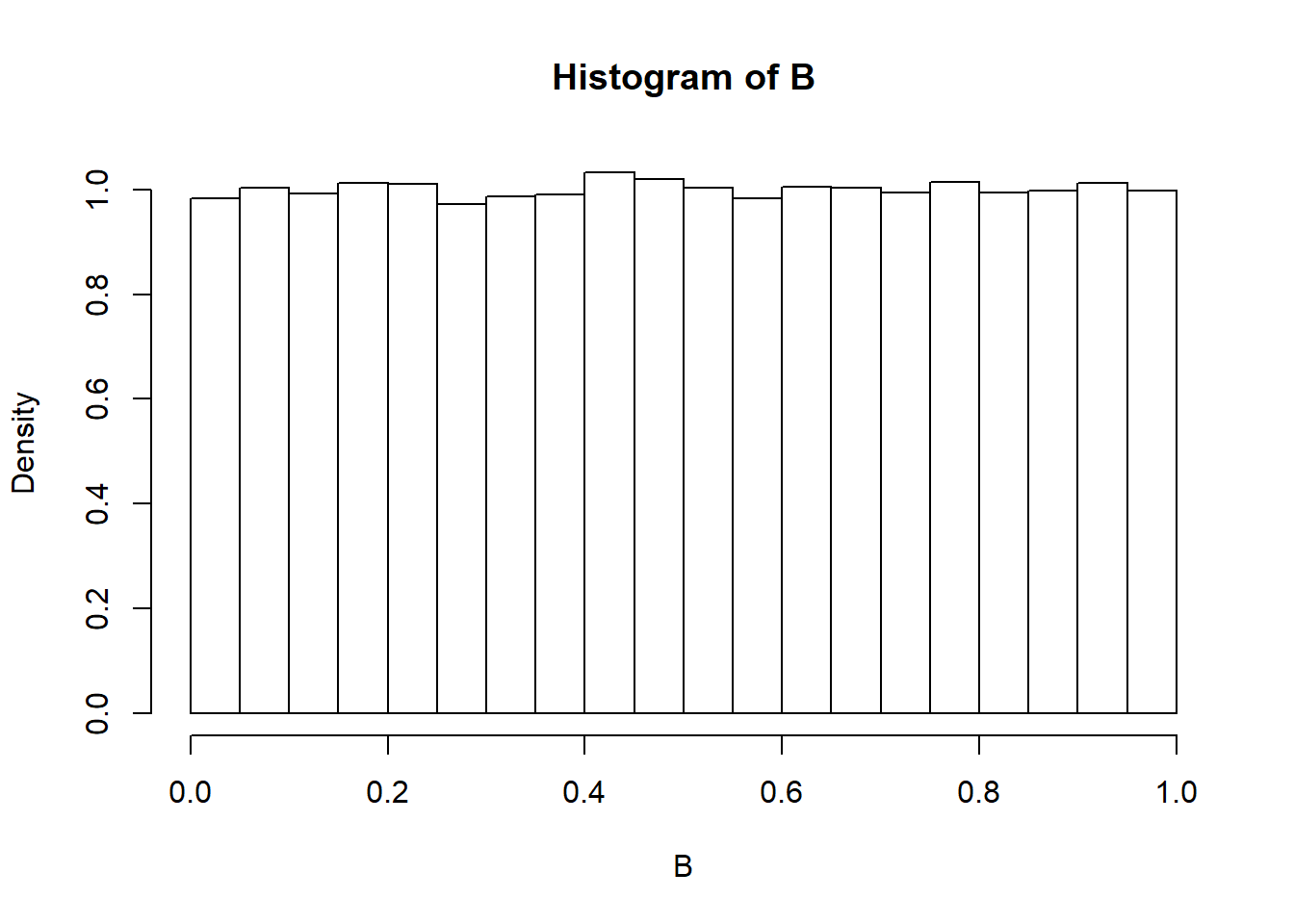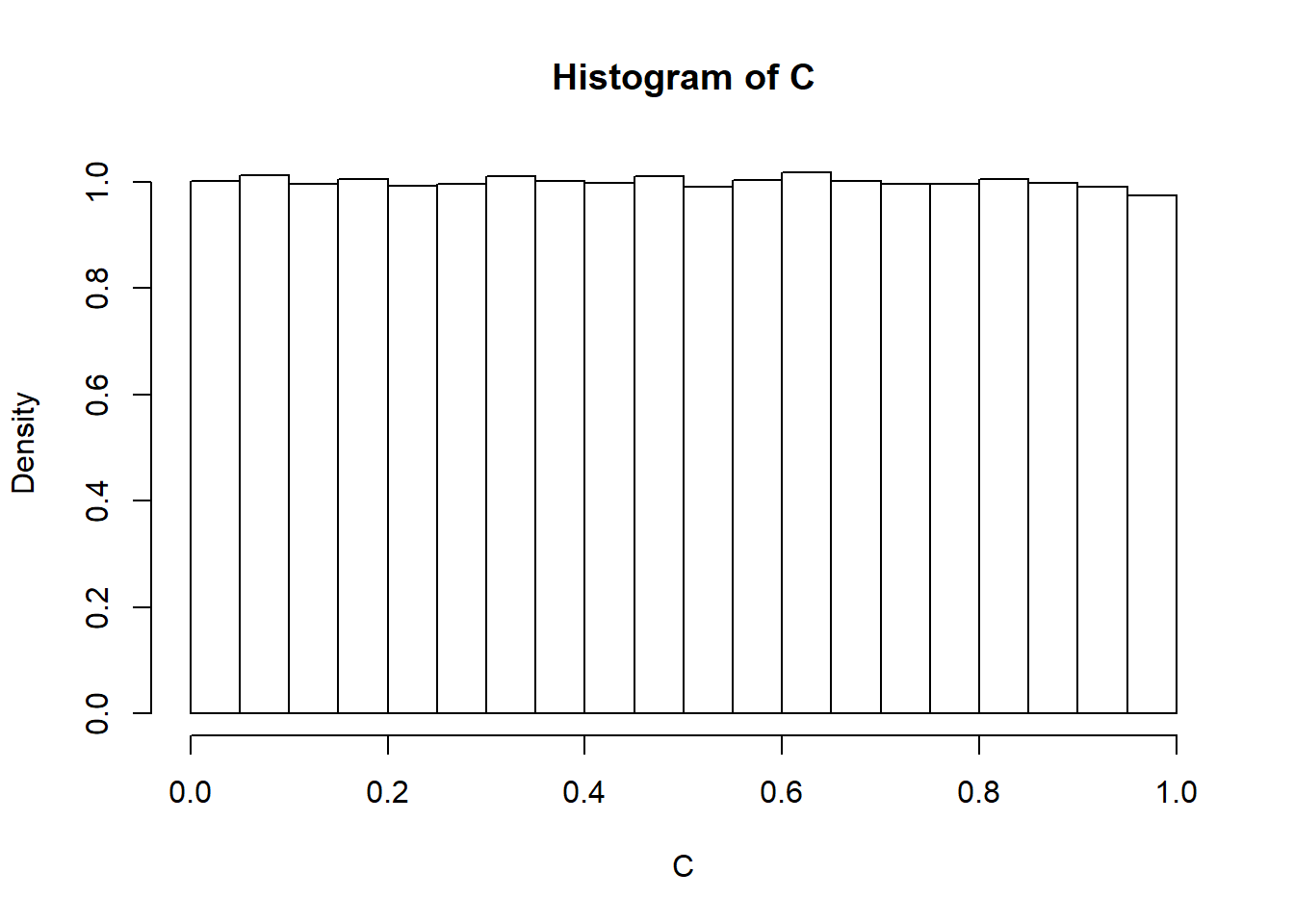## Choose independently two numbers B and C at random from the interval [0, 1] with uniform density. Prove that B and C are proper probability distributions. Note that the point (B,C) is then chosen at random in the unit square. Find the probability that:

# We simulate 100000 trials for selection of number B from the interval 0 to 1, with equal probability of each outcome

B<-runif(100000, min=0, max=1) 
# We check the minimum and maximum values for the number B
min(B)
##  1.081359e-05
max(B)
##  0.9999895

We see that all the values are non-zero

hist(B, probability = TRUE)The histogram of values of B shows that the density of all values adds up to 1. So both the conditions of a probability distribution are satisfied. The function is positive everywhere and the cumulative values add up to 1.

We repeat the same calculations for function C and arrive at the same conclusion as well.

C<-runif(100000, min=0, max=1)
min(C)
##  1.039822e-06
max(C)
##  0.9999814
hist(C, probability = TRUE)## Find the probability that:

1. B + C < 1/2
2. BC < 1/2
3. |B ??? C| < 1/2
4. max{B,C} < 1/2
5. min{B,C} < 1/2
compound1<-B+C
sum((compound1)<.5)/100000
##  0.12516
compound2<-B*C
sum((compound2)<.5)/100000
##  0.84705
compound3<-abs(B-C)
sum((compound3)<.5)/100000
##  0.75099
compound4<-pmax(B,C)
sum((compound4)<.5)/100000
##  0.25041
compound5<-pmin(B,C)
sum((compound5)<.5)/100000
##  0.7509